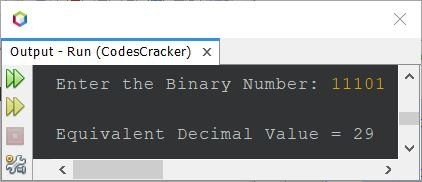# Java Program to Convert Binary to Decimal

This article covers a program in Java that coverts binary to decimal number. That is, a binary number entered by user at run-time of the program, gets converted into its equivalent decimal value.

Note - If you're not aware about, how the binary to decimal conversion takes place, then refer to Binary to Decimal Conversion. Now let's move and create the program.

## Binary to Decimal in Java using while Loop

The question is, write a Java program to convert binary to decimal. The binary number must be received by user at run-time. The program given below is its answer:

```import java.util.Scanner;

public class CodesCracker
{
public static void main(String[] args)
{
int binnum, decnum=0, i=1, rem;
Scanner scan = new Scanner(System.in);

System.out.print("Enter the Binary Number: ");
binnum = scan.nextInt();

while(binnum!=0)
{
rem = binnum%10;
decnum = decnum + (rem*i);
i = i*2;
binnum = binnum/10;
}

System.out.println("\nEquivalent Decimal Value = " +decnum);
}
}```

The snapshot given below shows the sample run of above Java program, on converting binary number to decimal number, with user input 11101:## Binary to Decimal in Java using for Loop

This is the same program as of previous, but creating using for loop, instead of while.

```import java.util.Scanner;

public class CodesCracker
{
public static void main(String[] args)
{
int binnum, decnum=0, i=1, rem;
Scanner scan = new Scanner(System.in);

System.out.print("Enter the Binary Number: ");
binnum = scan.nextInt();

for(i=1; binnum!=0; binnum /= 10)
{
rem = binnum%10;
decnum = decnum + (rem*i);
i *= 2;
}

System.out.println("\nEquivalent Decimal Value = " +decnum);
}
}```

You'll get the same output as of previous program. To increase the limit, you can use long instead of int data type. The range of int is 2,147,483,647, whereas the range of long is 9,223,372,036,854,775,807.

#### Same Program in Other Languages

Java Online Test

« Previous Program Next Program »